Home > A2C > Chapter 7 > Lesson 7.1.2 > Problem7-27

7-27.
1. Flipping a penny and a nickel can be shown in a tree diagram. Use the tree diagram at right to calculate the following probabilities. Homework Help ✎

1. P(H, T )

2. (Note: This means the probability of getting one head and then one tail.)

3. P(T, H )

4. P(H, H) or P(T, T )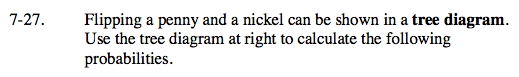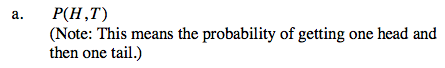Note that it says one head and then one tail, meaning that the head has to come first, and the tail second.

$\frac{1}{4}$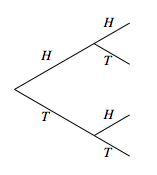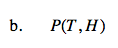See part (a).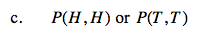Is the probability of either event happening more likely or less likely than one event happening exclusively?